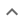•# Adding, subtracting and finding the least common multiple

We think you wrote:

###This solution deals with adding, subtracting and finding the least common multiple.

Solution foundt=±sqrt(3)=±1.7321

## Step by Step Solution### Rearrange:

Rearrange the equation by subtracting what is to the right of the equal sign from both sides of the equation :

2/3*t-(t-1/t)=0

## Step  1  :

            1
Simplify   —
t


#### Equation at the end of step  1  :

   2               1
(— • t) -  (t -  —)  = 0
3               t


## Step  2  :

#### Rewriting the whole as an Equivalent Fraction :

Subtracting a fraction from a whole

Rewrite the whole as a fraction using  t  as the denominator :

          t     t • t
t =  —  =  —————
1       t


Equivalent fraction : The fraction thus generated looks different but has the same value as the whole

Common denominator : The equivalent fraction and the other fraction involved in the calculation share the same denominator

#### Adding fractions that have a common denominator :

Adding up the two equivalent fractions
Add the two equivalent fractions which now have a common denominator

Combine the numerators together, put the sum or difference over the common denominator then reduce to lowest terms if possible:

 t • t - (1)     t2 - 1
———————————  =  ——————
t            t


#### Equation at the end of step  2  :

   2         (t2 - 1)
(— • t) -  ————————  = 0
3            t


## Step  3  :

            2
Simplify   —
3


#### Equation at the end of step  3  :

   2         (t2 - 1)
(— • t) -  ————————  = 0
3            t


## Step  4  :

#### Trying to factor as a Difference of Squares :

Factoring:  t2-1

Theory : A difference of two perfect squares,  A2 - B2  can be factored into  (A+B) • (A-B)

Proof :  (A+B) • (A-B) =
A2 - AB + BA - B2 =
A2 - AB + AB - B2 =
A2 - B2

Note :  AB = BA is the commutative property of multiplication.

Note :  - AB + AB equals zero and is therefore eliminated from the expression.

Check : 1 is the square of 1
Check :  t2  is the square of  t1

Factorization is :       (t + 1)  •  (t - 1)

#### Calculating the Least Common Multiple :

Find the Least Common Multiple

The left denominator is :       3

The right denominator is :       t

Number of times each prime factor
appears in the factorization of:
Prime
Factor
Left
Denominator
Right
Denominator
L.C.M = Max
{Left,Right}
3101
Product of all
Prime Factors
313

Number of times each Algebraic Factor
appears in the factorization of:
Algebraic
Factor
Left
Denominator
Right
Denominator
L.C.M = Max
{Left,Right}
t 011

Least Common Multiple:
3t

#### Calculating Multipliers :

Calculate multipliers for the two fractions

Denote the Least Common Multiple by  L.C.M
Denote the Left Multiplier by  Left_M
Denote the Right Multiplier by  Right_M
Denote the Left Deniminator by  L_Deno
Denote the Right Multiplier by  R_Deno

Left_M = L.C.M / L_Deno = t

Right_M = L.C.M / R_Deno = 3

#### Making Equivalent Fractions :

Rewrite the two fractions into equivalent fractions

Two fractions are called equivalent if they have the same numeric value.

For example :  1/2   and  2/4  are equivalent,  y/(y+1)2   and  (y2+y)/(y+1)3  are equivalent as well.

To calculate equivalent fraction , multiply the Numerator of each fraction, by its respective Multiplier.

   L. Mult. • L. Num.      2t • t
——————————————————  =   ——————
L.C.M               3t

R. Mult. • R. Num.      (t+1) • (t-1) • 3
——————————————————  =   —————————————————
L.C.M                    3t


#### Adding fractions that have a common denominator :

Adding up the two equivalent fractions

 2t • t - ((t+1) • (t-1) • 3)     3 - t2
————————————————————————————  =  ——————
3t                    3t


#### Trying to factor as a Difference of Squares :

Factoring:  3 - t2

Check :  3  is not a square !!

Ruling : Binomial can not be factored as the
difference of two perfect squares

#### Equation at the end of step  4  :

  3 - t2
——————  = 0
3t


## Step  5  :

#### When a fraction equals zero :

 5.1    When a fraction equals zero ...

Where a fraction equals zero, its numerator, the part which is above the fraction line, must equal zero.

Now,to get rid of the denominator, Tiger multiplys both sides of the equation by the denominator.

Here's how:

  3-t2
———— • 3t = 0 • 3t
3t


Now, on the left hand side, the  3t  cancels out the denominator, while, on the right hand side, zero times anything is still zero.

The equation now takes the shape :
3-t2  = 0

#### Solving a Single Variable Equation :

Solve  :    -t2+3 = 0

Subtract  3  from both sides of the equation :

-t2 = -3
Multiply both sides of the equation by (-1) :  t2 = 3

When two things are equal, their square roots are equal. Taking the square root of the two sides of the equation we get:

t  =  ± √ 3

The equation has two real solutions

These solutions are  t = ± √3 = ± 1.7321

## Two solutions were found :

t = ± √3 = ± 1.7321